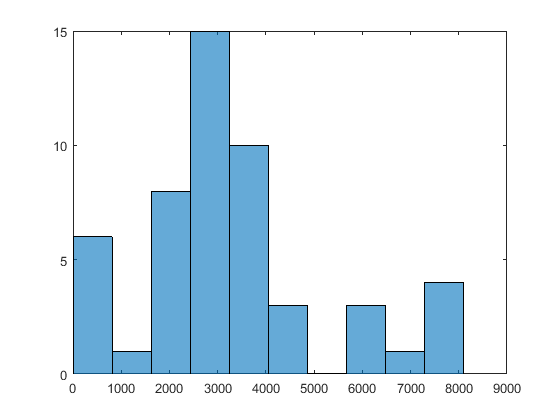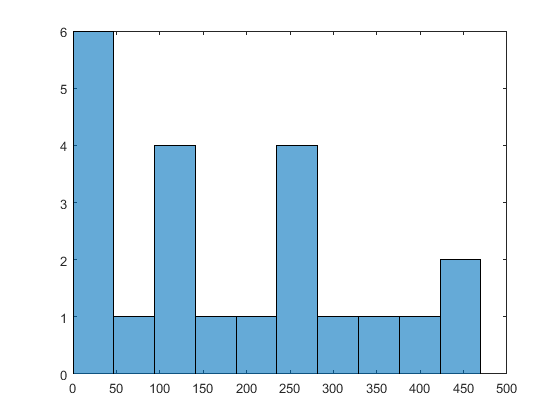## Refine Start Points

If some components of your problem are unconstrained, `GlobalSearch` and `MultiStart` use artificial bounds to generate random start points uniformly in each component. However, if your problem has far-flung minima, you need widely dispersed start points to find these minima.

Use these methods to obtain widely dispersed start points:

• Give widely separated bounds in your `problem` structure.

• Use a `RandomStartPointSet` object with the `MultiStart` algorithm. Set a large value of the `ArtificialBound` property in the `RandomStartPointSet` object.

• Use a `CustomStartPointSet` object with the `MultiStart` algorithm. Use widely dispersed start points.

Give bounds in `problem`Automatic point generationMakes a more complex Hessian
Can use with `GlobalSearch`Unclear how large to set the bounds
Easy to doChanges `problem`
Bounds can be asymmetric Only uniform points
Large `ArtificialBound` in `RandomStartPointSet`Automatic point generation`MultiStart` only
Does not change `problem`Only symmetric, uniform points
Easy to doUnclear how large to set `ArtificialBound`
`CustomStartPointSet`Customizable`MultiStart` only
Does not change `problem`Requires programming for generating points

### Methods of Generating Start Points

#### Uniform Grid

To generate a uniform grid of start points:

1. Generate multidimensional arrays with `ndgrid`. Give the lower bound, spacing, and upper bound for each component.

For example, to generate a set of three-dimensional arrays with

• First component from –2 through 0, spacing 0.5

• Second component from 0 through 2, spacing 0.25

• Third component from –10 through 5, spacing 1

`[X,Y,Z] = ndgrid(-2:.5:0,0:.25:2,-10:5);`
2. Place the arrays into a single matrix, with each row representing one start point. For example:

`W = [X(:),Y(:),Z(:)];`

In this example, `W` is a 720-by-3 matrix.

3. Put the matrix into a `CustomStartPointSet` object. For example:

`custpts = CustomStartPointSet(W);`

Call `run` with the `CustomStartPointSet` object as the third input. For example,

```% Assume problem structure and ms MultiStart object exist [x,fval,flag,outpt,manymins] = run(ms,problem,custpts);```

#### Perturbed Grid

Integer start points can yield less robust solutions than slightly perturbed start points.

To obtain a perturbed set of start points:

1. Generate a matrix of start points as in steps 1–2 of Uniform Grid.

2. Perturb the start points by adding a random normal matrix with 0 mean and relatively small variance.

For the example in Uniform Grid, after making the `W` matrix, add a perturbation:

```[X,Y,Z] = ndgrid(-2:.5:0,0:.25:2,-10:5); W = [X(:),Y(:),Z(:)]; W = W + 0.01*randn(size(W));```
3. Put the matrix into a `CustomStartPointSet` object. For example:

`custpts = CustomStartPointSet(W);`

Call `run` with the `CustomStartPointSet` object as the third input. For example,

```% Assume problem structure and ms MultiStart object exist [x,fval,flag,outpt,manymins] = run(ms,problem,custpts);```

#### Widely Dispersed Points for Unconstrained Components

Some components of your problem can lack upper or lower bounds. For example:

• Although no explicit bounds exist, there are levels that the components cannot attain. For example, if one component represents the weight of a single diamond, there is an implicit upper bound of 1 kg (the Hope Diamond is under 10 g). In such a case, give the implicit bound as an upper bound.

• There truly is no upper bound. For example, the size of a computer file in bytes has no effective upper bound. The largest size can be in gigabytes or terabytes today, but in 10 years, who knows?

For truly unbounded components, you can use the following methods of sampling. To generate approximately 1/n points in each region (exp(n),exp(n+1)), use the following formula. If u is random and uniformly distributed from 0 through 1, then r = 2u – 1 is uniformly distributed between –1 and 1. Take

`$y=\mathrm{sgn}\left(r\right)\left(\mathrm{exp}\left(1/|r|\right)-e\right).$`

y is symmetric and random. For a variable bounded below by `lb`, take

`$y=\text{lb}+\left(\mathrm{exp}\left(1/u\right)-e\right).$`

Similarly, for a variable bounded above by `ub`, take

`$y=\text{ub}-\left(\mathrm{exp}\left(1/u\right)-e\right).$`

For example, suppose you have a three-dimensional problem with

• `x`(1) > 0

• `x`(2) < 100

• `x`(3) unconstrained

To make 150 start points satisfying these constraints:

```u = rand(150,3); r1 = 1./u(:,1); r1 = exp(r1) - exp(1); r2 = 1./u(:,2); r2 = -exp(r2) + exp(1) + 100; r3 = 1./(2*u(:,3)-1); r3 = sign(r3).*(exp(abs(r3)) - exp(1)); custpts = CustomStartPointSet([r1,r2,r3]);```

The following is a variant of this algorithm. Generate a number between 0 and infinity by the method for lower bounds. Use this number as the radius of a point. Generate the other components of the point by taking random numbers for each component and multiply by the radius. You can normalize the random numbers, before multiplying by the radius, so their norm is 1. For a worked example of this method, see MultiStart Without Bounds, Widely Dispersed Start Points.

### Example: Searching for a Better Solution

`MultiStart` fails to find the global minimum in Multiple Local Minima Via MultiStart. There are two simple ways to search for a better solution:

• Use more start points

• Give tighter bounds on the search space

Set up the problem structure and `MultiStart` object:

```problem = createOptimProblem('fminunc',... 'objective',@(x)sawtoothxy(x(1),x(2)),... 'x0',[100,-50],'options',... optimoptions(@fminunc,'Algorithm','quasi-newton')); ms = MultiStart;```

#### Use More Start Points

Run `MultiStart` on the problem for 200 start points instead of 50:

```rng(14,'twister') % for reproducibility [x,fval,eflag,output,manymins] = run(ms,problem,200) MultiStart completed some of the runs from the start points. 53 out of 200 local solver runs converged with a positive local solver exit flag. x = 1.0e-06 * -0.2284 -0.5567 fval = 2.1382e-12 eflag = 2 output = struct with fields: funcCount: 32670 localSolverTotal: 200 localSolverSuccess: 53 localSolverIncomplete: 147 localSolverNoSolution: 0 message: 'MultiStart completed some of the runs from the start points.↵↵53 out of 200 local solver runs converged with a positive local solver exit flag.' manymins = 1x53 GlobalOptimSolution Properties: X Fval Exitflag Output X0```

This time `MultiStart` found the global minimum, and found 51 local minima.

To see the range of local solutions, enter `histogram([manymins.Fval],10)`.#### Tighter Bound on the Start Points

Suppose you believe that the interesting local solutions have absolute values of all components less than 100. The default value of the bound on start points is 1000. To use a different value of the bound, generate a `RandomStartPointSet` with the `ArtificialBound` property set to `100`:

```startpts = RandomStartPointSet('ArtificialBound',100,... 'NumStartPoints',50); [x,fval,eflag,output,manymins] = run(ms,problem,startpts) MultiStart completed some of the runs from the start points. 29 out of 50 local solver runs converged with a positive local solver exit flag. x = 1.0e-08 * 0.9725 -0.6198 fval = 1.4955e-15 eflag = 2 output = struct with fields: funcCount: 7431 localSolverTotal: 50 localSolverSuccess: 29 localSolverIncomplete: 21 localSolverNoSolution: 0 message: 'MultiStart completed some of the runs from the start points.↵↵29 out of 50 local solver runs converged with a positive local solver exit flag.' manymins = 1x25 GlobalOptimSolution Properties: X Fval Exitflag Output X0```

`MultiStart` found the global minimum, and found 22 distinct local solutions. To see the range of local solutions, enter `histogram([manymins.Fval],10)`.Compared to the minima found in Use More Start Points, this run found better (smaller) minima, and had a higher percentage of successful runs.

Watch now